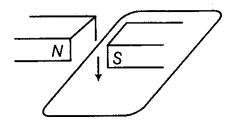# The wire in the figure below is being moved downwards through the magnetic field

The wire in the figure below is being moved downwards through the magnetic field so as to produce induced current.What would be the effect of
(i) moving the wire at a higher speed?
(ii) moving the wire upwards rather than downwards?
(iii) using a stronger magnet?
(iv) holding the wire still in the magnetic field?

(i) The induced current increases at a higher speed.
(ii) The induced current is reversed.
(iii) The induced current increases.
(iv) The induced current is zero.